Pattern Completion Quiz III, Non Verbal Reasoning

# Pattern Completion Quiz III, Non Verbal Reasoning - SSC CGL

Test Description

## 25 Questions MCQ Test Non Verbal Reasoning - Pattern Completion Quiz III, Non Verbal Reasoning

Pattern Completion Quiz III, Non Verbal Reasoning for SSC CGL 2023 is part of Non Verbal Reasoning preparation. The Pattern Completion Quiz III, Non Verbal Reasoning questions and answers have been prepared according to the SSC CGL exam syllabus.The Pattern Completion Quiz III, Non Verbal Reasoning MCQs are made for SSC CGL 2023 Exam. Find important definitions, questions, notes, meanings, examples, exercises, MCQs and online tests for Pattern Completion Quiz III, Non Verbal Reasoning below.
Solutions of Pattern Completion Quiz III, Non Verbal Reasoning questions in English are available as part of our Non Verbal Reasoning for SSC CGL & Pattern Completion Quiz III, Non Verbal Reasoning solutions in Hindi for Non Verbal Reasoning course. Download more important topics, notes, lectures and mock test series for SSC CGL Exam by signing up for free. Attempt Pattern Completion Quiz III, Non Verbal Reasoning | 25 questions in 50 minutes | Mock test for SSC CGL preparation | Free important questions MCQ to study Non Verbal Reasoning for SSC CGL Exam | Download free PDF with solutions
 1 Crore+ students have signed up on EduRev. Have you?
Pattern Completion Quiz III, Non Verbal Reasoning - Question 1

### Directions to Solve In each of the following questions, select a figure from amongst the four alternatives, which when placed in the blank space of figure (X) would complete the pattern. Question - Identify the figure that completes the pattern.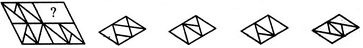(X)                 (1)             (2)              (3)             (4)

Detailed Solution for Pattern Completion Quiz III, Non Verbal Reasoning - Question 1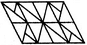Pattern Completion Quiz III, Non Verbal Reasoning - Question 2

### Directions to Solve In each of the following questions, select a figure from amongst the four alternatives, which when placed in the blank space of figure (X) would complete the pattern. Question - Identify the figure that completes the pattern.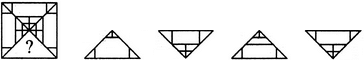(X)                 (1)             (2)              (3)             (4)

Detailed Solution for Pattern Completion Quiz III, Non Verbal Reasoning - Question 2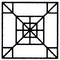Pattern Completion Quiz III, Non Verbal Reasoning - Question 3

### Directions to Solve In each of the following questions, select a figure from amongst the four alternatives, which when placed in the blank space of figure (X) would complete the pattern. Question - Identify the figure that completes the pattern.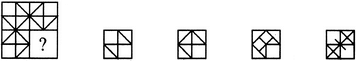(X)                 (1)             (2)              (3)             (4)

Detailed Solution for Pattern Completion Quiz III, Non Verbal Reasoning - Question 3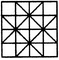Pattern Completion Quiz III, Non Verbal Reasoning - Question 4

In each of the following questions, select a figure from amongst the four alternatives, which when placed in the blank space of figure (X) would complete the pattern.

Question -

Identify the figure that completes the pattern.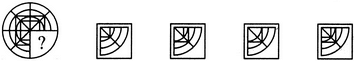(X)                 (1)             (2)              (3)             (4)
Detailed Solution for Pattern Completion Quiz III, Non Verbal Reasoning - Question 4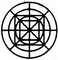Pattern Completion Quiz III, Non Verbal Reasoning - Question 5

In each of the following questions, select a figure from amongst the four alternatives, which when placed in the blank space of figure (X) would complete the pattern.

Question -

Identify the figure that completes the pattern.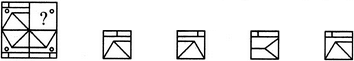(X)                 (1)             (2)              (3)             (4)
Detailed Solution for Pattern Completion Quiz III, Non Verbal Reasoning - Question 5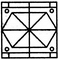Pattern Completion Quiz III, Non Verbal Reasoning - Question 6

In each of the following questions, select a figure from amongst the four alternatives, which when placed in the blank space of figure (X) would complete the pattern.

Question -

Identify the figure that completes the pattern.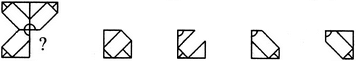(X)                 (1)             (2)              (3)             (4)
Detailed Solution for Pattern Completion Quiz III, Non Verbal Reasoning - Question 6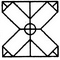Pattern Completion Quiz III, Non Verbal Reasoning - Question 7

In each of the following questions, select a figure from amongst the four alternatives, which when placed in the blank space of figure (X) would complete the pattern.

Question -

Identify the figure that completes the pattern.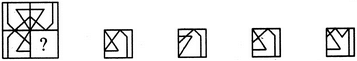(X)                 (1)             (2)              (3)             (4)
Detailed Solution for Pattern Completion Quiz III, Non Verbal Reasoning - Question 7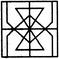Pattern Completion Quiz III, Non Verbal Reasoning - Question 8

In each of the following questions, select a figure from amongst the four alternatives, which when placed in the blank space of figure (X) would complete the pattern.

Question -

Identify the figure that completes the pattern.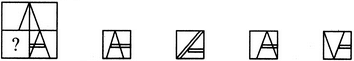(X)                 (1)             (2)              (3)             (4)
Detailed Solution for Pattern Completion Quiz III, Non Verbal Reasoning - Question 8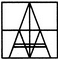Pattern Completion Quiz III, Non Verbal Reasoning - Question 9

In each of the following questions, select a figure from amongst the four alternatives, which when placed in the blank space of figure (X) would complete the pattern.

Question -

Identify the figure that completes the pattern.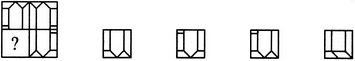(X)                 (1)             (2)              (3)             (4)
Detailed Solution for Pattern Completion Quiz III, Non Verbal Reasoning - Question 9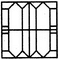Pattern Completion Quiz III, Non Verbal Reasoning - Question 10

In each of the following questions, select a figure from amongst the four alternatives, which when placed in the blank space of figure (X) would complete the pattern.

Question -

Identify the figure that completes the pattern.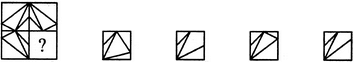(X)                 (1)             (2)              (3)             (4)
Detailed Solution for Pattern Completion Quiz III, Non Verbal Reasoning - Question 10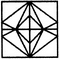Pattern Completion Quiz III, Non Verbal Reasoning - Question 11

In each of the following questions, select a figure from amongst the four alternatives, which when placed in the blank space of figure (X) would complete the pattern.

Question -

Identify the figure that completes the pattern.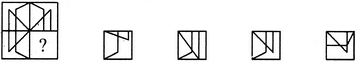(X)                 (1)             (2)              (3)             (4)
Detailed Solution for Pattern Completion Quiz III, Non Verbal Reasoning - Question 11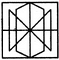Pattern Completion Quiz III, Non Verbal Reasoning - Question 12

In each of the following questions, select a figure from amongst the four alternatives, which when placed in the blank space of figure (X) would complete the pattern.

Question -

Identify the figure that completes the pattern.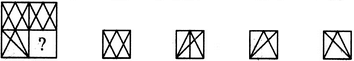(X)                 (1)             (2)              (3)             (4)
Detailed Solution for Pattern Completion Quiz III, Non Verbal Reasoning - Question 12Pattern Completion Quiz III, Non Verbal Reasoning - Question 13

In each of the following questions, select a figure from amongst the four alternatives, which when placed in the blank space of figure (X) would complete the pattern.

Question -

Identify the figure that completes the pattern.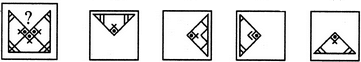(X)                 (1)             (2)              (3)             (4)
Detailed Solution for Pattern Completion Quiz III, Non Verbal Reasoning - Question 13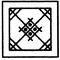Pattern Completion Quiz III, Non Verbal Reasoning - Question 14

In each of the following questions, select a figure from amongst the four alternatives, which when placed in the blank space of figure (X) would complete the pattern.

Question -

Identify the figure that completes the pattern.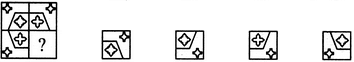(X)                 (1)             (2)              (3)             (4)
Detailed Solution for Pattern Completion Quiz III, Non Verbal Reasoning - Question 14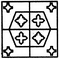Pattern Completion Quiz III, Non Verbal Reasoning - Question 15

In each of the following questions, select a figure from amongst the four alternatives, which when placed in the blank space of figure (X) would complete the pattern.

Question -

Identify the figure that completes the pattern.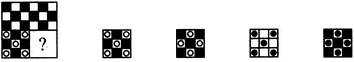(X)                 (1)             (2)              (3)             (4)
Detailed Solution for Pattern Completion Quiz III, Non Verbal Reasoning - Question 15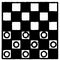Pattern Completion Quiz III, Non Verbal Reasoning - Question 16

In each of the following questions, select a figure from amongst the four alternatives, which when placed in the blank space of figure (X) would complete the pattern.

Question -

Identify the figure that completes the pattern.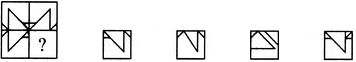(X)                 (1)             (2)              (3)             (4)
Detailed Solution for Pattern Completion Quiz III, Non Verbal Reasoning - Question 16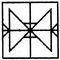Pattern Completion Quiz III, Non Verbal Reasoning - Question 17

In each of the following questions, select a figure from amongst the four alternatives, which when placed in the blank space of figure (X) would complete the pattern.

Question -

Identify the figure that completes the pattern.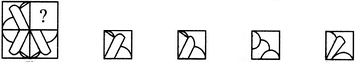(X)                 (1)             (2)              (3)             (4)
Detailed Solution for Pattern Completion Quiz III, Non Verbal Reasoning - Question 17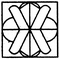Pattern Completion Quiz III, Non Verbal Reasoning - Question 18

In each of the following questions, select a figure from amongst the four alternatives, which when placed in the blank space of figure (X) would complete the pattern.

Question -

Identify the figure that completes the pattern.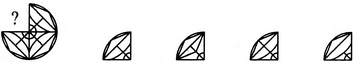(X)                 (1)             (2)              (3)             (4)
Detailed Solution for Pattern Completion Quiz III, Non Verbal Reasoning - Question 18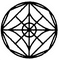Pattern Completion Quiz III, Non Verbal Reasoning - Question 19

In each of the following questions, select a figure from amongst the four alternatives, which when placed in the blank space of figure (X) would complete the pattern.

Question -

Identify the figure that completes the pattern.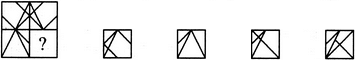(X)                 (1)             (2)              (3)             (4)
Detailed Solution for Pattern Completion Quiz III, Non Verbal Reasoning - Question 19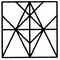Pattern Completion Quiz III, Non Verbal Reasoning - Question 20

In each of the following questions, select a figure from amongst the four alternatives, which when placed in the blank space of figure (X) would complete the pattern.

Question -

Identify the figure that completes the pattern.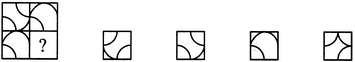(X)                 (1)             (2)              (3)             (4)
Detailed Solution for Pattern Completion Quiz III, Non Verbal Reasoning - Question 20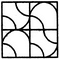Pattern Completion Quiz III, Non Verbal Reasoning - Question 21

In each of the following questions, select a figure from amongst the four alternatives, which when placed in the blank space of figure (X) would complete the pattern.

Question -

Identify the figure that completes the pattern.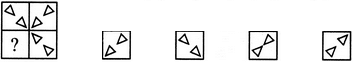(X)                 (1)             (2)              (3)             (4)
Detailed Solution for Pattern Completion Quiz III, Non Verbal Reasoning - Question 21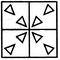Pattern Completion Quiz III, Non Verbal Reasoning - Question 22

In each of the following questions, select a figure from amongst the four alternatives, which when placed in the blank space of figure (X) would complete the pattern.

Question -

Identify the figure that completes the pattern.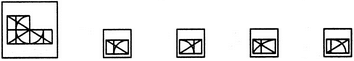(X)                 (1)             (2)              (3)             (4)
Detailed Solution for Pattern Completion Quiz III, Non Verbal Reasoning - Question 22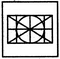Pattern Completion Quiz III, Non Verbal Reasoning - Question 23

In each of the following questions, select a figure from amongst the four alternatives, which when placed in the blank space of figure (X) would complete the pattern.

Question -

Identify the figure that completes the pattern.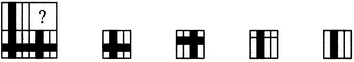(X)                 (1)             (2)              (3)             (4)
Detailed Solution for Pattern Completion Quiz III, Non Verbal Reasoning - Question 23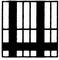Pattern Completion Quiz III, Non Verbal Reasoning - Question 24

In each of the following questions, select a figure from amongst the four alternatives, which when placed in the blank space of figure (X) would complete the pattern.

Question -

Identify the figure that completes the pattern.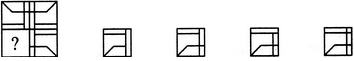(X)                 (1)             (2)              (3)             (4)
Detailed Solution for Pattern Completion Quiz III, Non Verbal Reasoning - Question 24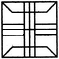Pattern Completion Quiz III, Non Verbal Reasoning - Question 25

In each of the following questions, select a figure from amongst the four alternatives, which when placed in the blank space of figure (X) would complete the pattern.

Question -

Identify the figure that completes the pattern.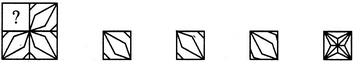(X)                 (1)             (2)              (3)             (4)
Detailed Solution for Pattern Completion Quiz III, Non Verbal Reasoning - Question 25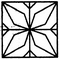## Non Verbal Reasoning

40 tests
Information about Pattern Completion Quiz III, Non Verbal Reasoning Page
In this test you can find the Exam questions for Pattern Completion Quiz III, Non Verbal Reasoning solved & explained in the simplest way possible. Besides giving Questions and answers for Pattern Completion Quiz III, Non Verbal Reasoning, EduRev gives you an ample number of Online tests for practice

40 tests